# Java核心基础第4篇-Java数组的常规操作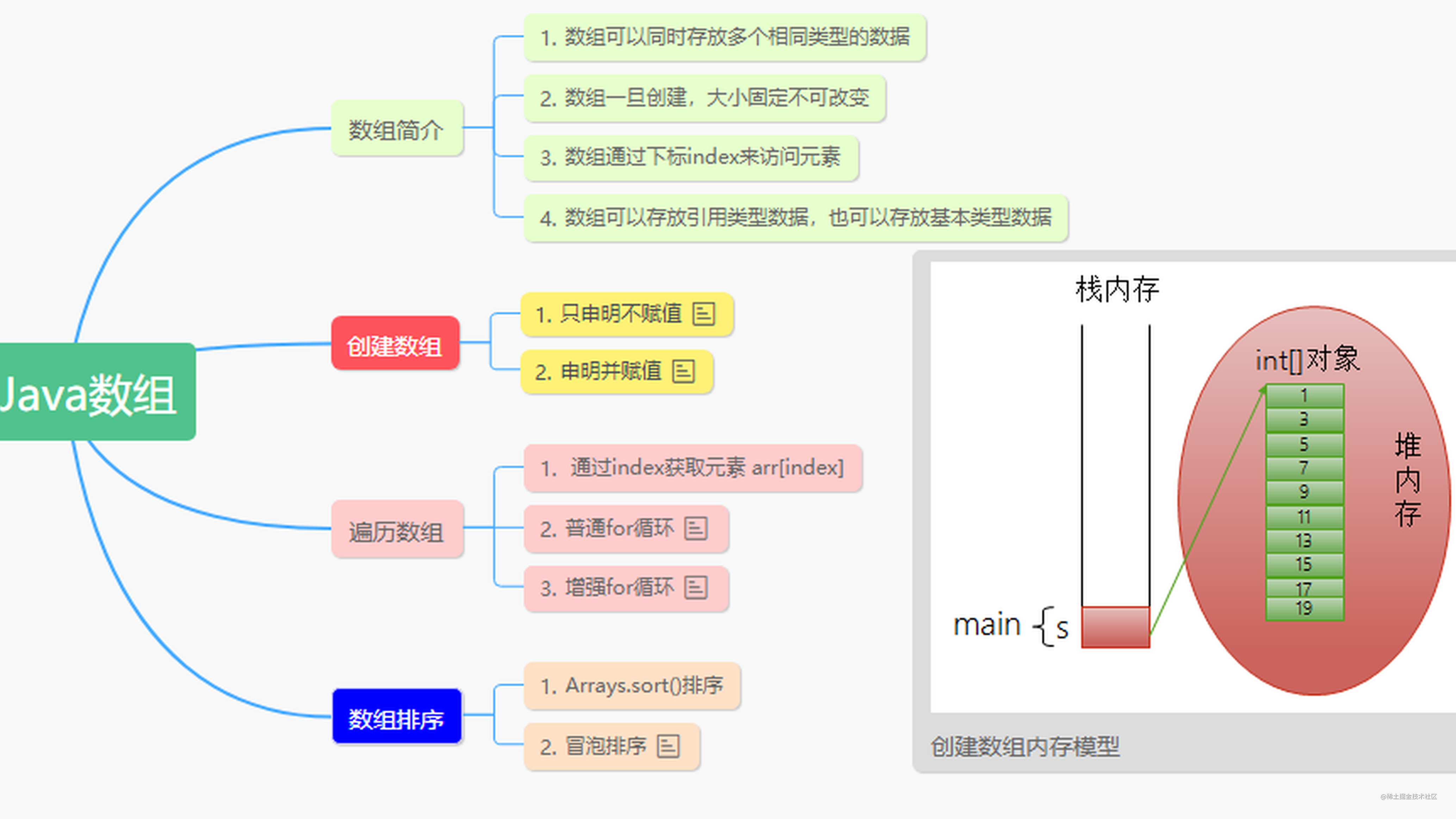# Java数组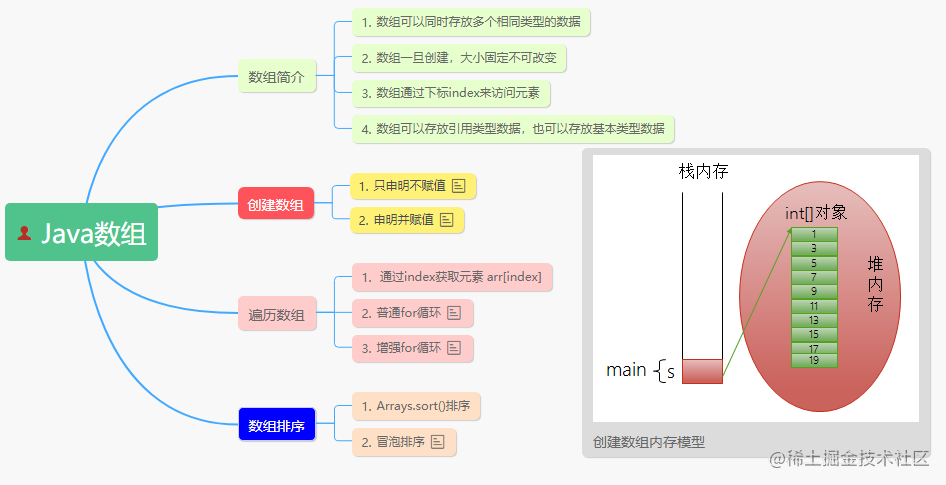# 一、数组简介

• 数组是多个相同类型数据的组合，实现对这些数据的统一管理
• 数组属引用类型，数组型数据是对象(Object)
• 数组中的元素可以是任何数据类型，包括基本类型和引用类型
• 数组类型是单一，只能存放相同类型的数据
• 数组一旦创建，大小就是固定的
• 数组中每个元素都有自己的位置(下标),从0开始

``````int scores = {100,90,98,99,88,80}

# 二、创建数组

`int [] a``int a[]`含义是一样的，`[]``变量名`前后是没有关系的。

### 2.1 只申明不赋值

type [] var;或者 type var [] ;

``````int score[];
int [] score;
Object [] obj;  //Object是java语法中老祖宗

### 2.2 申明并赋值

type [] var = new type[size]; //创建的是数据，数组的元素为空。值默认的就是该类型的默认值

type [] var = {elm,elem2,elem3,...} //创建的同时，把数据也赋值了

``````public static void main(String[] args) {

// 申明 默认值
int score[] = new int;
for(int i = 0;i<score.length;i++) {
System.out.print(score[i]+"\t");
}

System.out.println("------------");
//创建并且赋值
int score2[] = {100,90,88,85,66};
for(int i = 0;i<score2.length;i++) {
System.out.print(score2[i]+"\t");
}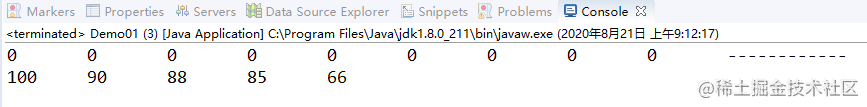### 2.3 new数组内存模型

new:java中的关键字，主要作用用来在内存中，开辟新的空间，从而创建新的对象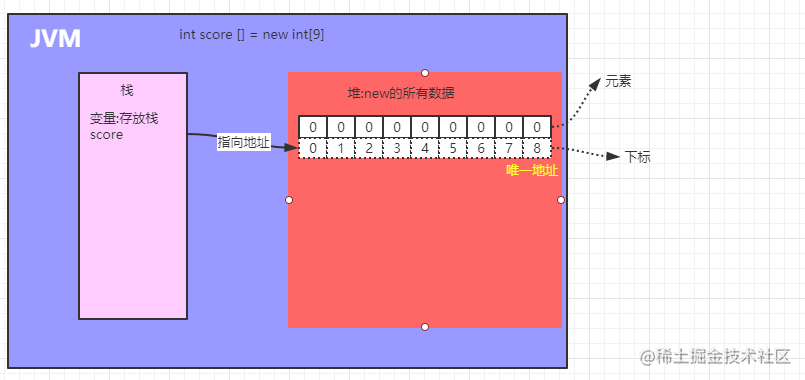### 2.4 下标操作元素

#### 2.4.1 通过下标给元素赋值

``````int score[] = new int;

//指定元素赋值
score = 10;
score = 50;

#### 2.4.2 通过下标获取元素内容

``````int num2 = score;
System.out.println("num2="+num2);

for(int i = 0;i<score.length;i++) {
System.out.print(score[i]+"\t");
}

### 2.5 注意事项

• Java中使用关键字 new 创建数组对象
• 定义并用运算符 new 为之分配空间后，才可以引用数组中的每个元素；
• 数组元素的引用方式：数组名[数组元素下标]
• 数组元素下标可以是整型常量或整型表达式。如a , b[i] , c[6*i];
• 数组元素下标从0开始；长度为n的数组合法下标取值范围: 0 - n-1；如int a[]=new int; 可引用的数组元素为a、a、a
• 每个数组都有一个属性 length 指明它的长度，例如：a.length 指明数组 a 的长度(元素个数)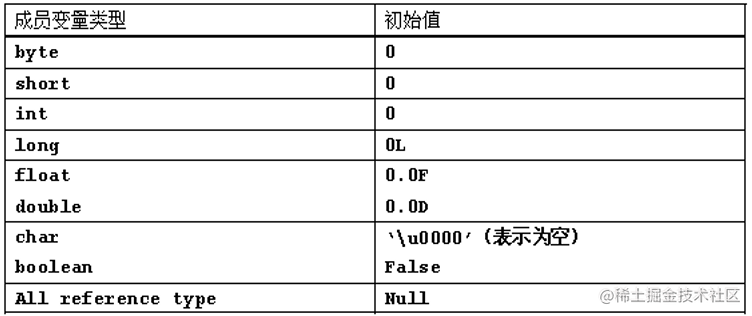# 三、遍历数组

## 3.1 普通for循环

``````int score[] = {100,90,88,85,66};
for(int i = 0;i<score.length;i++) {
System.out.println(score2[i]);
}

## 3.2 增强for循环

Java有一种功能很强的循环结构，可以用来依次处理数组中的每个元素而不必为指定下标值而分心。 这种增强的for循环的语句格式为：

`for(variable : arr)statement`

``````int score[] = {100,90,88,85,66};
for(int ele:score){
System.out.println(ele);
}

# 四、数组拷贝

``````int score[] = {100, 90, 88, 85, 66};

//长度超过原数据的部分：使用默认0替代
int[] newArr01 = Arrays.copyOf(score, 10);

//长度不足的，从前往后取对应的数据
int[] newArr02 = Arrays.copyOf(score, 3);

System.out.println("原数组 = " + Arrays.toString(score));
System.out.println("新数组1 = " + Arrays.toString(newArr01));
System.out.println("新数组2 = " + Arrays.toString(newArr02));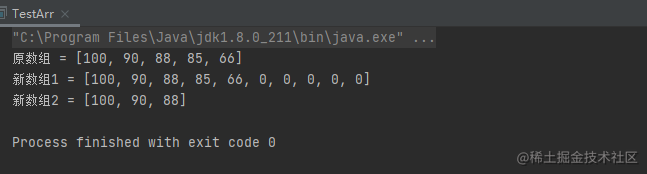• 如果数组元素是数值型，那么多余的元素将被赋值为0；
• 如果数组元素是布尔型，则将赋值为false。
• 相反，如果长度小于原始数组的长度，则只拷贝最前面的数据元素。

# 五、数组排序

### 5.1 Arrays工具类排序

java.util.Arrays：属于工具类，所以在使用之前，必须先导包

``````public static void main(String[] args) {
int score [] = {99,89,78,88,69,70};
String ch[] = {"B","C","A","F","D"};
System.out.println("排序前:");
for (int i = 0; i < ch.length; i++) {
System.out.print(ch[i]+"\t");
}
System.out.println();

//进行排序
//1.只提供了升序排序 从小到大  没有提供降序。自己去实现
//2.也叫做自然顺序。 A(65) B(66) C(67) D(68)
Arrays.sort(ch);

System.out.println("排序后:");
for (int i = 0; i < ch.length; i++) {
System.out.print(ch[i]+"\t");
}
}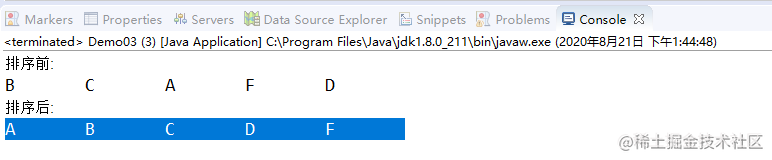### 4.2 通过算法实现排序(冒泡)

1. 将相邻的两个数，进行比较，将大的数据往后移动。依次循环进行比较，从而找到最大的数据
2. 由于每一次循环只能确定一个数据，所以需要多次嵌套循环来完成最终的排序
3. 详细如下图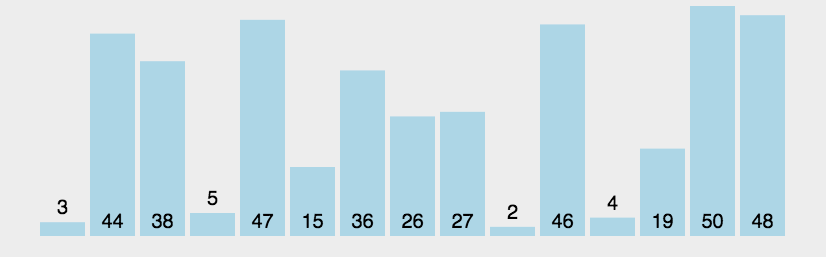``````public static void main(String[] args) {

int score [] = {99,89,78,88,69,70};
//i=0 {89,78,88,69,70,99};
//i=1 {78,88,69,70,89,99};
//i=2 {78,69,70,88,89,99};

System.out.println("第原始的结果:");
for (int j = 0; j < score.length; j++) {
System.out.print(score[j]+"\t");
}
System.out.println();

//排序：把前面几次排好了，最后一个自动确定了位置 。少排序一次
//每次选一个最大的
for (int i = 0; i < score.length-1; i++) {

//相邻的两个进行比较，把大的往后移
for (int j = 0; j < score.length-1-i; j++) {
//如果说 前面比后面的大，两者更换位置
if(score[j]>score[j+1]) {
//两两互换，通过临时变量
int temp = score[j];
score[j] = score[j+1];
score[j+1] = temp;
}

}
System.out.println("第"+(i+1)+"次执行的结果:");
for (int j = 0; j < score.length; j++) {
System.out.print(score[j]+"\t");
}
System.out.println();
}
}